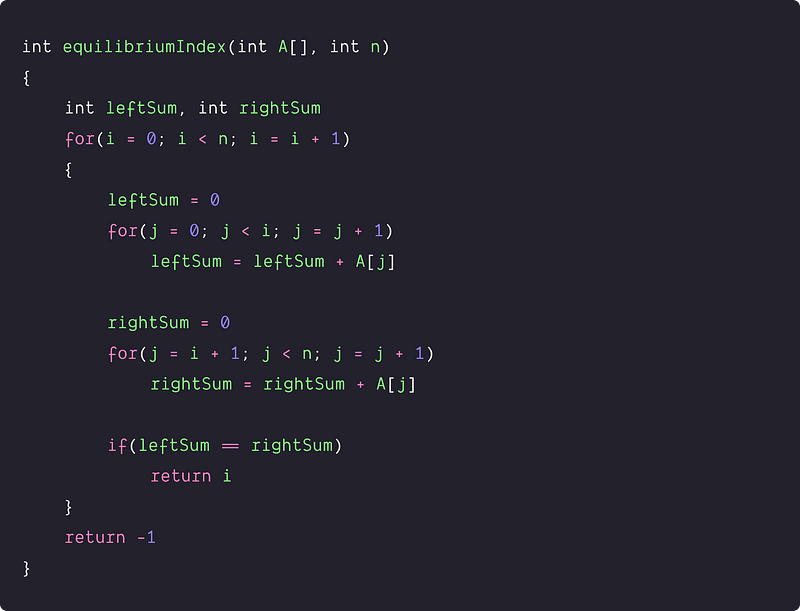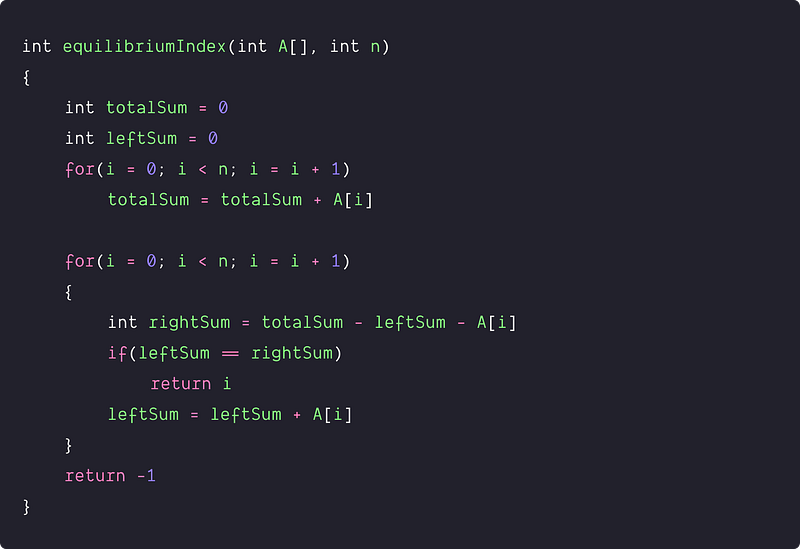# Find equilibrium index of an array

Key Takeaway: Learn problem-solving and optimization using a single loop.

### Let’s understand the problem

Write a program to find the equilibrium index of an array. The equilibrium index of an array is an index such that the sum of elements at lower indexes is equal to the sum of elements at higher indexes. In other words, the equilibrium index of an array is an index i such that the sum of elements at indices less than i is equal to the sum of elements at indices greater than i.

A + A+….. + A[i-1] = A[i+1] + ….. + A[n-1], Where 0 < i < n-1

Examples

Input: A[] = [-7, 1, 5, 2, -4, 3, 0], Output: 3

Explanation: 3 is an equilibrium index A + A + A = A + A + A

Input: A[] = [0, 1, 3, -2, -1], Output: 1

Explanation: 1 is an equilibrium index, A = A + A + A

### Follow-up questions for the interviewer

• Candidate: For calculating the equilibrium index, Do we need to include index i in the left or right part? Interviewer: No, the element at index i is not included in either part.
• Candidate: Is there a possibility of a multiple equilibrium index? Interviewer: No, It is stated that at max, there is only one equilibrium index in the array. Return the index if it is present; otherwise, return -1.
• Candidate: Are the input values only positive? Interviewer: No, It may be positive, negative, or zero.
• Candidate: Are the input values unique? Interviewer: No, it can be repeated.

### Discussed solution approaches

• A brute force approach using two nested loops
• Using extra space: Prefix sum array
• An efficient approach using a single loop

### A brute force approach using two nested loops

Solution Idea

A straightforward method is to use two loops. The idea is to find the sum of elements for every range of indexes and observe if there exists an equilibrium index. The outer loop traverses the array, and the inner loop determines if there is an equilibrium index or not. For this, we can use two extra variables:

• leftSum: the sum of all elements which is left of the current index
• rightSum: the sum of all elements which is right of the current index

Solution Steps

• Declare variables leftSum and righSum
• Run a loop through the array from 0 to n-1. For each index i, find the sum of elements towards the left and right of the current index. If the leftSum and rightSum are equal, then the current index is the equilibrium index.
• return -1 by end of the loop because we did not find the equilibrium index.

Solution PseudocodeTime and space complexity analysis

We are running nested loops and calculating leftSum and rightSum for every value of i. Total number of summation operation = n(n-1) (Think!) Time complexity = O(n²), Space complexity: O(1)

### Using extra space: Prefix sum array

Solution Idea

The idea is to store the prefix sum of the array. The prefix sum would help keep track of the sum of all elements up to any index. Now, we should find a way to keep track of the sum of values to the right of the current index. We can use a temporary sum variable, which initially stores the sum of all elements of the array. If we subtract the value at the current index, we will get the sum of values to the right. Now, compare both leftSum and rightSum to find whether the current index is the equilibrium index or not.

Solution Steps

• Declare prefix sum array of size n
• Scan the array using loop and store prefix sum of the array
``````for(i = 0; i < n; i = i + 1)
{
if(i == 0)
prefix[i] = A[i]
else
prefix[i] = A[i] + prefix[i-1]
}
``````
• Get the total sum of the array in variable totalSum i.e totalSum = prefix[n-1]
• Iterate through the array and calculate variables leftSum and rightSum. If we subtract prefix[i] from the value at current index i, we will get the value of leftSum. Similarly, if If we subtract totalSum from prefix[i], we will get the value of rightSum. Now, compare both leftSum and rightSum to find whether the current index is the equilibrium index or not. If leftSum equals rightSum, return the current index i.
``````for(i = 0; i < n; i = i + 1)
{
int leftSum = prefix[i] - A[i]
int rightSum = totalSum - prefix[i]
if(leftSum == rightSum)
return i
}
``````
• return -1 by end of the loop because we did not find the equilibrium index.

Solution PseudocodeTime and space complexity analysis

We are running two separate loops for calculating the prefix sum array and equilibrium index respectively. Time Complexity = Time complexity of calculating prefix sum array + Time complexity of calculating Equilibrium Index = O(n) + O(n) = O(n)

Space Complexity: The above algorithm uses extra space to create a prefix sum array. Hence, the space complexity would be O(n).

### An efficient approach using a single loop

Solution Idea

The motive is to get the total sum of the array first. The difference between the above method and this method is that we don’t need to store the prefix sum beforehand. Instead, we will keep track of the leftSum and rightSum while traversing the array itself.

Solution Steps

• Declare and initialize the variables totalSum and leftSum.
• Now run a loop to store the total sum of the array in variable totalSum
``````for(i = 0; i < n; i = i + 1)
totalSum = totalSum + A[i]
``````
• Iterate through the array and keep track of the variables leftSum and rightSum. Now, compare both leftSum and rightSum to find whether the current index is the equilibrium index or not. If leftSum equals rightSum, return the current index i.
``````for(i = 0; i < n; i = i + 1)
{
int rightSum = totalSum - leftSum - A[i]
if(leftSum == rightSum)
return i
leftSum = leftSum + A[i]
}
``````
• return -1 by end of the loop because we did not find the equilibrium index.

Solution PseudocodeTime and space complexity analysis

We are running two separate loops for calculating the total sum and equilibrium index respectively. Time complexity = Time complexity of calculating prefix sum array + Time complexity of calculating Equilibrium Index = O(n) + O(n) = O(n). Space complexity = O(1), we are using variables to store the value of total, left, and the right sum.

### Critical ideas to think!

• Can we solve this problem using the hash table?
• Can we solve this problem using two pointer approach?
• How to modify the above code if there can be multiple equilibrium indexes? What would be the different corner cases?
• In the efficient approach, why are calculating leftSum after the comparison if(leftSum == rightSum)?

### Comparisons of time and space complexities

• Brute force approach: Time = O(n²), Space = O(1)
• Using prefix sum array: Time = O(n), Space = O(n)
• Using single loop: Time = O(n), Space = O(1)

### Suggested coding questions to practice

Thanks to Navtosh Kumar for his contribution in creating the first version of the content. Please write comments if you find an error or you want to share more insights about the topic. Enjoy learning, Enjoy coding, Enjoy algorithms!

Get well-designed application and interview centirc content on ds-algorithms, machine learning, system design and oops. Content will be delivered weekly.